﻿ 层间接触条件下粘弹性铺装层蠕变分析 Creep Analysis of Viscoelastic Pavement under Interlayer Contact Condition

International Journal of Mechanics Research
Vol. 09  No. 01 ( 2020 ), Article ID: 34118 , 9 pages
10.12677/IJM.2020.91001

Creep Analysis of Viscoelastic Pavement under Interlayer Contact Condition

Xiang Chen, Hu Wang, Ya Wang

School of Science, Chang’an University, Xi’an Shaanxi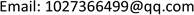Received: Jan. 15th, 2020; accepted: Jan. 30th, 2020; published: Feb. 6th, 2020ABSTRACT

In order to analyze the creep response of the viscoelastic pavement layer during interlayer contact, a finite element analysis model was established by simplifying the upper structure of the bridge, and the creep law of the pavement layer was analyzed under different load forms, different temperatures and different contact cohesion. The results show that the settlement displacement of asphalt layer under impact load is basically the same as that under vertical load, but it will produce large vertical uplift displacement, and its creep deformation is more complicated. The larger of the influence of loading time on creep of asphalt surface layer, and the higher the temperature, the greater the deformation, the faster the creep stability period; the temperature mainly affects the vertical ridge displacement of the surface layer, and the vertical bulge displacement increases with the increase of temperature, i.e. when the temperature is high, the unstable rut is more likely to occur; under the long-term loading of the impact load, when the cohesive force is 0.01 MPa, local slip occurs between the layers; when the cohesive force is greater than 0.01 MPa, the creep response of the asphalt surface layer remains the same.

Keywords:Bridge Deck Pavement, Interlayer Contact, Viscoelasticity, Creep, Finite Element Analysis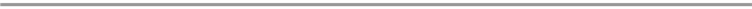1. 引言

2. 沥青的粘弹性模型

2.1. 基本理论

Burgers模型的本构方程可写为

$\sigma +{p}_{1}\stackrel{˙}{\sigma }+{p}_{2}\stackrel{¨}{\sigma }={q}_{1}\stackrel{˙}{\epsilon }+{q}_{2}\stackrel{¨}{\epsilon }$ (1)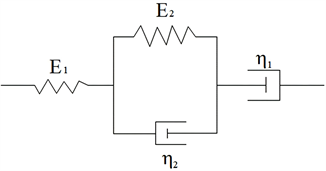Figure 1. Burgers modelTable 1. Parameters of Burgers model of asphalt materials

2.2. 参数转换

Burgers模型参数不能直接作为prony级数的输入量，需要将其转化为prony级数的剪切模量表达式中所对应的参数。由prony级数来表征粘弹性材料的属性时，其基本形式如下：

$G\left(t\right)={G}_{\infty }+\underset{i=1}{\overset{n}{\sum }}{G}_{i}\mathrm{exp}\left(-\frac{t}{{\tau }_{i}}\right)$ (2)

${\alpha }_{i}=\frac{{G}_{i}}{{G}_{0}}$ (3)

${G}_{1}=\frac{{E}_{1}}{2\left(1+{\mu }_{1}\right)},\text{}{G}_{2}=\frac{{E}_{2}}{2\left(1+{\mu }_{2}\right)},\text{}{n}_{1}=\frac{{\eta }_{1}}{2\left(1+{\mu }_{1}\right)},\text{}{n}_{2}=\frac{{\eta }_{2}}{2\left(1+{\mu }_{2}\right)},\text{}{\mu }_{1}={\mu }_{2}$ (4)

${p}_{1}=\frac{{n}_{1}}{{G}_{1}}+\frac{{n}_{1}+{n}_{2}}{{G}_{2}},\text{\hspace{0.17em}}\text{\hspace{0.17em}}{p}_{2}=\frac{{n}_{1}{n}_{2}}{{G}_{1}{G}_{2}},\text{\hspace{0.17em}}\text{\hspace{0.17em}}{q}_{1}=2{n}_{1},\text{\hspace{0.17em}}\text{\hspace{0.17em}}{q}_{2}=\frac{2{n}_{1}{n}_{2}}{{G}_{2}}$ (5)

$\begin{array}{c}G\left(t\right)=\frac{{G}_{1}}{\alpha -\beta }\left[\left(\frac{{G}_{2}}{{n}_{2}}-\beta \right)\cdot \mathrm{exp}\left(-\beta t\right)+\left(\alpha -\frac{{G}_{2}}{{n}_{2}}\right)\cdot \mathrm{exp}\left(-\alpha t\right)\right]\\ ={G}_{0}\left[{\alpha }_{\infty }+{\alpha }_{1}\mathrm{exp}\left(-\frac{t}{{\tau }_{1}}\right)+{\alpha }_{2}\mathrm{exp}\left(-\frac{t}{{\tau }_{2}}\right)\right]\end{array}$ (6)

${G}_{0}={G}_{1},\text{\hspace{0.17em}}\text{\hspace{0.17em}}{\alpha }_{\infty }=0,\text{\hspace{0.17em}}\text{\hspace{0.17em}}{\alpha }_{1}=\frac{1}{\alpha -\beta }\left(\frac{{G}_{2}}{{n}_{2}}-\beta \right),\text{\hspace{0.17em}}\text{\hspace{0.17em}}{\tau }_{1}=\frac{1}{\beta },\text{\hspace{0.17em}}\text{\hspace{0.17em}}{\alpha }_{2}=\frac{1}{\alpha -\beta }\left(\alpha -\frac{{G}_{2}}{{n}_{2}}\right),\text{\hspace{0.17em}}\text{\hspace{0.17em}}{\tau }_{2}=\frac{1}{\alpha }$Table 2. Prony series parameters for asphalt materials

3. 有限元模型建立

3.1. 层间接触设置

${\tau }_{\mathrm{lim}}=\mu P+b$ (7)

$|\tau |\le {\tau }_{\mathrm{lim}}$ (8)

3.2. 模型参数及荷载布置

3.2.1. 模型参数Table 3. Material parameters of waterproof adhesive layer

3.2.2. 荷载布置

$F=0.5G$ (9)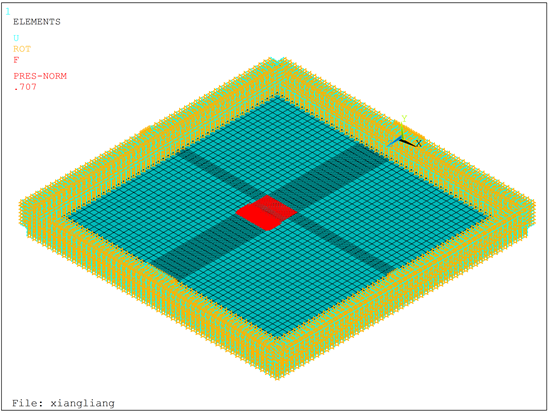Figure 2. Finite element model

4. 铺装层蠕变规律分析

4.1. 沥青面层在不同荷载下的变化响应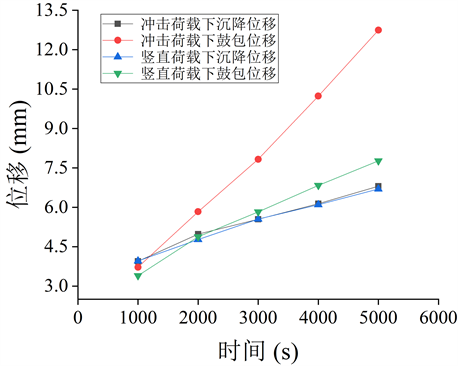Figure 3. Time history curve of extreme point displacement under different loads

4.2. 沥青层蠕变随时间变化

4.3. 沥青层蠕变随温度变化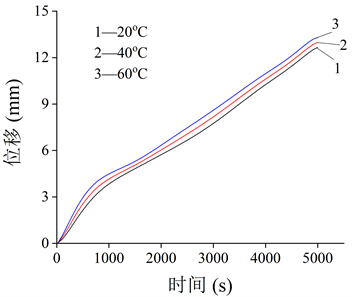Figure 4. Time history curve of bulged displacement of asphalt surface layer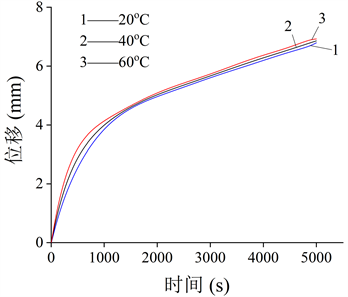Figure 5. Settlement displacement time history curve of asphalt surface layer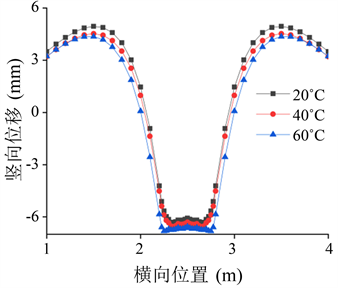Figure 6. Lateral joint displacement at different temperatures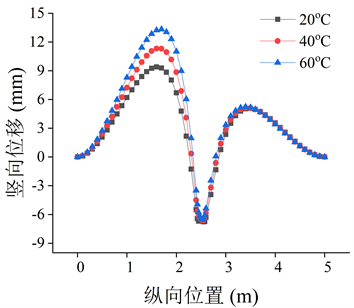Figure 7. Displacement of longitudinal joints at different temperatures

4.4. 沥青层蠕变随层间粘聚力变化Table 4. Creep response under different cohesion forces

5. 结论

1) 在冲击荷载与竖直荷载的长时间作用下，沥青层的蠕变变形略有不同，冲击荷载下会使得沥青层剪应力过大而产生较大的竖向隆起位移，其产生的变形更为复杂。

2) 加载时间的长短对沥青面层蠕变影响较大，沥青面层经受长时间荷载累积后，便会产生永久性变形；温度越高，产生的蠕变变形越大，进入蠕变稳定期更快，更易产生永久变形。

3) 在冲击荷载作用下，会在荷载作用区的行车方向周围产生较大竖向位移，且温度对其的影响较大，即高温时更易出现失稳型车辙。

4) 当黏聚力为0.01 MPa时，在冲击荷载的长时间加载下，层间出现部分滑移；当黏聚力大于0.01 MPa时，其力学响应保持不变。

Creep Analysis of Viscoelastic Pavement under Interlayer Contact Condition[J]. 力学研究, 2020, 09(01): 1-9. https://doi.org/10.12677/IJM.2020.91001

1. 1. 侯曙光. 基于动态蠕变试验的沥青混合料黏弹性分析[J]. 南京工业大学学报(自然科学版), 2010, 32(1): 33-36.

2. 2. 董满生, 鹿婧, 凌天清, 等. 考虑温度效应的沥青混合料参数模型[J]. 工程力学, 2016, 33(6): 180-185, 193.

3. 3. 万晨光, 申爱琴, 郭寅川, 等. 层间接触状态对桥面铺装结构力学响应的影响[J]. 江苏大学学报(自然科学版), 2016, 37(2): 236-241.

4. 4. 汪凡. 基于流变学本构模型和动力有限元分析的沥青路面车辙计算[D]: [硕士学位论文]. 重庆: 重庆交通大学, 2009.

5. 5. 叶青. 基于粘弹性的沥青混合料疲劳性能研究[D]: [硕士学位论文]. 哈尔滨: 哈尔滨工业大学, 2016.

6. 6. 曹卫锋, 吕彭民. 粘弹性沥青路面车辆动力响应仿真分析研究[J]. 计算机仿真, 2013, 30(4): 173-177.

7. 7. 龙尧, 谢晶, 王德群, 等. 基于Burgers模型的沥青混合料室内车辙试验粘弹性分析[J]. 中外公路, 2011, 31(5): 239-242.

8. 8. 陈静云, 周长红, 王哲人. 沥青混合料蠕变试验数据处理与粘弹性计算[J]. 东南大学学报(自然科学版), 2007, 37(6): 1091-1095.

9. 9. 王琨, 郝培文. 沥青高温蠕变变形的粘弹性组成研究[J]. 公路工程, 2017, 42(5): 122-126, 172.

10. 10. 潘晓明, 余俊, 杨钊, 等. 一种将线性粘弹微分型本构方程应用到ABAQUS的方法[J]. 华侨大学学报(自然科学版), 2010, 31(5): 570-575.

11. 11. 王勋涛, 封建湖, 王虎. 层间接触时空心板桥铺装层应力分析[J]. 公路, 2017, 62(6): 14-22.

12. 12. 陈渊召. 沥青混合料桥面铺装新技术研究[M]. 北京: 中国水利水电出版社, 2016: 23-24.

13. 13. 中国建筑科学研究院. 混凝土结构设计规范GB50010-2015[S]. 北京: 中国建筑科学研究院, 2015.

14. 14. 胡钢. 层间接触状态对路面结构力学响应的影响[J]. 公路, 2015, 60(3): 34-40.